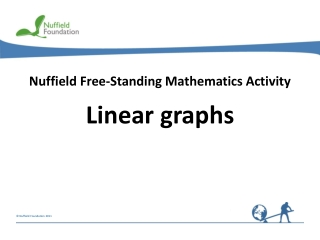DownloadDownload PresentationNuffield Free-Standing Mathematics Activity

# Nuffield Free-Standing Mathematics Activity

Download Presentation## Nuffield Free-Standing Mathematics Activity

- - - - - - - - - - - - - - - - - - - - - - - - - - - E N D - - - - - - - - - - - - - - - - - - - - - - - - - - -
##### Presentation Transcript

1. Nuffield Free-Standing Mathematics Activity Linear graphs

2. y x 0 Linear graphs • use an equation to calculate values In this activity you will: • plot graphs • look for a connection between the numbers in the equation and its graph

3. 5 4 3 2 1 –5 –4 –3 –2 –1 0 1 2 3 4 5 –1 –2 –3 –4 –5 A Coordinates x coordinate The position of a point is given by two coordinates. (2, 5) y coordinate y (2, 5) (– 2, 5 ) x (– 2, – 5 ) (2, – 5 )

4. x y 5 4 3 2 1 –5 –4 –3 –2 –1 0 1 2 3 4 5 –1 –2 –3 –4 –5 B Graphs of the form y = x + c 0 1 2 y = x + 2 2 3 4 y x y = x + 2

5. y y = x - 1 y = x - 2 5 4 3 2 x 1 –5 –4 –3 –2 –1 0 1 2 3 4 5 –1 y = x + 3 –2 y = x + 2 –3 y = x + 1 –4 –5 Answers for B

6. -1 0 1 2 x y y 5 4 3 2 1 x –5 –4 –3 –2 –1 0 1 2 3 4 5 –1 –2 –3 –4 –5 C Graphs of the form y = mx y = 3x -3 0 3 6 y = 3x

7. y = 2x y = -3x y = 3x y = -2x y 5 y = 0.5x 4 3 2 1 x –5 –4 –3 –2 –1 0 1 2 3 4 5 –1 –2 –3 –4 –5 Answers for C

8. 0 1 2 y = 2x - 1 x y y 5 4 3 2 1 x –5 –4 –3 –2 –1 0 1 2 3 4 5 –1 –2 –3 –4 –5 D Graphs of the formy = mx+ c - 1 1 3 y = 2x - 1

9. y y = 2x + 1 y = 2x - 1 5 4 3 y = 0.5x - 1 2 x 1 –5 –4 –3 –2 –1 0 1 2 3 4 5 –1 –2 –3 –4 –5 y = 3x - 1 Answers for D

10. Linear graphs Reflect on your work Explain to your partner what the values mandcstand for in the equation y=mx+c. For example, for the equation y =2x+ 1, what do the ‘2’ and the ‘1’ tell you about the graph?